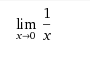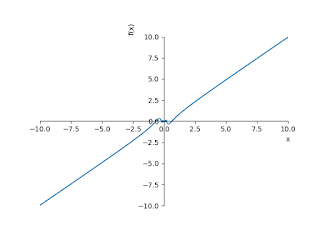## The limit of a function

In the case of Mathematics, The limit of a function is a fundamental concept in calculus. It analysis concerning the behavior of that function near a particular input.

Formal definitions, first formulated in the 19th century, are given below. Informally, a function f assigns an output f (x) to each input x. We say that the limit on the input L of the function is L: this means that f (x) moves closer to L and closer to x because x moves closer to P and moves. More specifically, when F is applied sufficiently close to P for any input, the output value is arbitrarily forced to be close to L.

On the other hand, if the input is moved too close to P for outputs that remain at a certain distance, then we say the limit does not exist.

The limit of a function has many applications in modern calculus. In particular, several definitions of continuity employ the limit: broadly, a function is continuous if all of its boundaries agree with the values of the function. It also appears in the definition of the derivative: in the algorithm of a variable, it is the finite value of the slope of the second lines in the graph of a function. has many applications in modern calculus. In particular, several definitions of continuity employ the limit: broadly, a function is continuous if all of its boundaries agree with the values of the function. It also appears in the definition of the derivative: in the algorithm of a variable, it is the finite value of the slope of the second lines in the graph of a function.

Let's take a look at Python code on how to plot the limit of a function as x tends to zero.

1. Discuss the Limit of the following function as x tends to zero.```import numpy as np
from sympy import *
x = symbols('x')
init_printing(use_unicode=True)
print(limit(1/x,x,0,'+'))
print(limit(1/x,x,0,'-'))
plot(1/x)```
```Output: oo
-oo``````
```
```
2. Discuss the Limit of the following function as x tends to zero.import numpy as np
from sympy import *
x = symbols('x')
init_printing(use_unicode=True)
print(limit(sin(1/x),x,0,'+'))
print(limit(sin(1/x),x,0,'-'))
plot(sin(1/x))
Output: AccumBounds(-1, 1)
AccumBounds(-1, 1)3. Discuss the Limit of the following function as x tends to zero.

``````import numpy as np
from sympy import *
x = symbols('x')
init_printing(use_unicode=True)
print(limit(cos(1/x),x,0,'+'))
print(limit(cos(1/x),x,0,'-'))
plot(cos(1/x))
Output: AccumBounds(-1, 1)
AccumBounds(-1, 1)4. Discuss the Limit of the following function as x tends to zero.import numpy as np
from sympy import *
x = symbols('x')
init_printing(use_unicode=True)
print(limit(x*sin(1/x),x,0,'+'))
print(limit(x*sin(1/x),x,0,'-'))
plot(x*sin(1/x))
Output: 05. Discuss the Limit of the following function as x tends to zero.import numpy as np
from sympy import *
x = symbols('x')
init_printing(use_unicode=True)
print(limit(x*cos(1/x),x,0,'+'))
print(limit(x*cos(1/x),x,0,'-'))
plot(x*cos(1/x))
Output:06. Discuss the Limit of the following function as x tends to zero.import numpy as np
from sympy import *
x = symbols('x')
init_printing(use_unicode=True)
print(limit(x**2*sin(1/x),x,0,'+'))
print(limit(x**2*sin(1/x),x,0,'-'))
plot(x**2*sin(1/x))
Output: 07. Discuss the Limit of the following function as x tends to zero.import numpy as np
from sympy import *
x = symbols('x')
init_printing(use_unicode=True)
print(limit(x**2*cos(1/x),x,0,'+'))
print(limit(x**2*cos(1/x),x,0,'-'))
plot(x**2*cos(1/x))
Output: 0```